The names we use determine how easily a concept clicks. Mathematicians struggled with the idea of a "negative" number. It's not there, but not zero either? It's somehow less than nothing?

You'll spin yourself dizzy trying to reconcile the ideas. So just rename it:

How about "forwards" and "backwards" numbers?

The invention of the number line vastly improved our understanding. What is "backwards from backwards"? It's forwards again! (I.e., why negative x negative = positive — more visual arithmetic.)

What is "halfway backwards"? Sideways!The wrong words limit our thoughts.

That this subject [imaginary numbers] has hitherto been surrounded by mysterious obscurity, is to be attributed largely to an ill adapted notation. If, for example, +1, -1, and the square root of -1 had been called direct, inverse and lateral units, instead of positive, negative and imaginary (or even impossible), such an obscurity would have been out of the question. - Carl Gauss

## Example: Counting

When we want a "count" of something, we aren't being specific enough. We're using too general a name.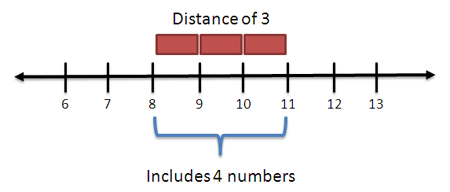There are two types of counts:

• The points that determine the boundaries
• The spans between the boundaries

Assuming there's a single, universal "count" sets ourselves up for off-by-one errors. Was that a "point count" or a "span count"?

"There are two hard things in computer science: cache invalidation, naming things, and off-by-one errors." - Phil Karlton

Even the name "off by one" isn't that helpful. That's the symptom, but what's the root cause? The "Fencepost error" helps identify why the issue happened.

## Example: Angles

How should we describe an angle? There's two ways to see it:

• Degrees, the swivel an observer went through to follow an object
• Radians, the distance the object moved on its path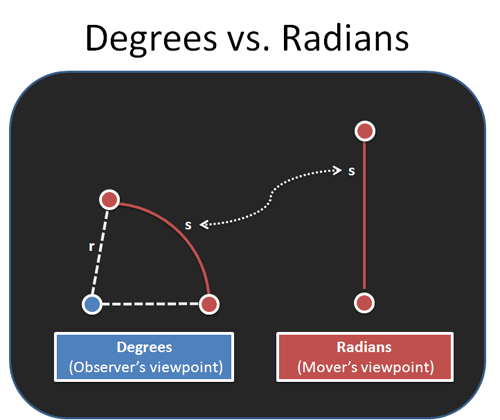When physics formulas (sine, cosine, etc.) ask for "angle in radians" they mean "distance the object moved". Aha!

(The laws of motion don't particularly care how much you, the observer, had to tilt your head. Sorry.)

## Example: Integrals

Integrals are usually described as the inverse of differentiation, finding the area under the curve, and so on.

That's it. You try to multiply two quantities, but you can't — one of the critters is scurrying around — so you use an integral.

"If I drive an unwavering 30mph for 3 hours, would just multiply it out. But since my speed changes, I'll integrate."

Think "fancy multiplication" not "inverse of differentiation". (Unless you're actually solving differential equations. In that case, have fun.)

## Example: The Pythagorean Theorem

Most formulas are named after their inventor. It's good to give credit, but it's not helpful for the student. For the Pythagorean Theorem, there's a few alternate names we can try:

• Triangle Theorem: Given two sides of a right triangle, we can know the third.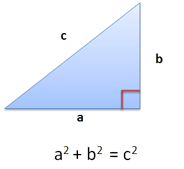• Distance Theorem: Get the distance between points in any number of dimensions. (By imagining they're on a sequence of triangles.)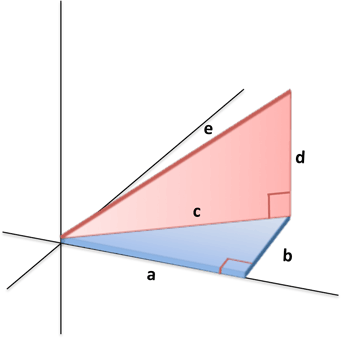• Tradeoff Theorem: Find the tradeoff as you move in any direction (how much "x distance" you give up to gain "y distance"). Using this, we can follow the gradient for the optimal direction.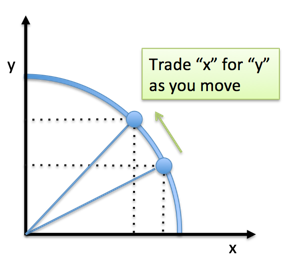Math gets so much easier with the right phrase in your head. Just use the "distance theorem" for distance, the "tradeoff theorem" to find the best direction to move.

Even if you forget the formula, you know how it's applied. Otherwise, you have the phrase "Pythagorean Theorem" without the understanding of why you'd need it.

## Example: The number e

A super-common question is "Why is the number e important"? It's all in the name.

e is the "universal growth constant" like c is the "speed of light constant". c is perfect speed (can't improve it further), e^x is perfect growth (can't compound it further).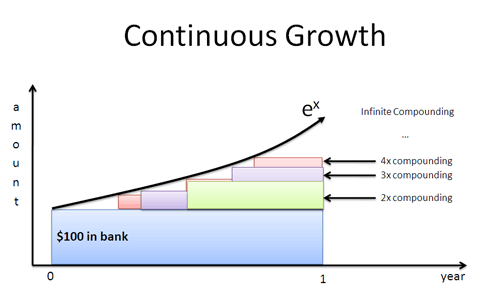e^x is what we see when compounding 100% with no delay. Why 100%? Symmetry, baby: our growth rate matches our current amount. (Similarly, we do trig on the unit circle, use 1.0 as our base increment for counting, and so on.)

Once we have perfection, we can modify it for our scenario. Maybe are growing for more time periods, or at a different rate — that's fine, just modify e^x.

Naming e "Euler's constant" isn't descriptive. The "universal growth constant" is more helpful.

## Example: Matrix Multiplication

I'm on a linear algebra kick. Instead of "Matrix multiplication" (which is a very drab description of what we're doing), how about "running data through operations" (or "running a spreadsheet").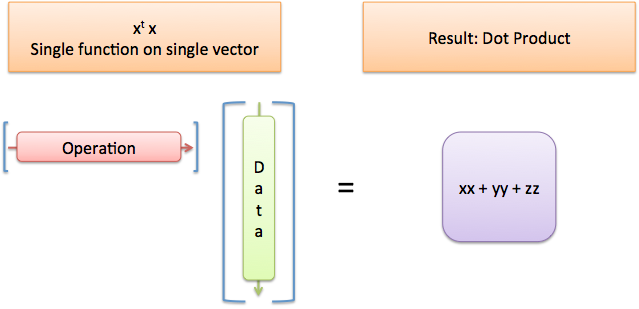One matrix represents the operations, one matrix represents the data, and we are running the data through a pipeline.

Yes, in some cases you can think about "linearly transforming a vector space" but often times we're just transforming data.

And if you have an error? Instead of "You're multiplying it wrong" (gee, thanks) how about "The operations expect different-sized data." Ah! I know what to fix.

## Make Your Own Names

They never tell you how much easier math gets when you create your own names for things. I use whatever analogies, metaphors, or plain-English descriptions help. They may feel silly, but it's a much better feeling than confusion.

There's an ancient concept that knowing the name of thing gives you power over it. It seems to be true for math.

## Appendix: Poincare on names

A famous mathematician had a great quote on naming:

"Mathematics is the art of giving the same name to different things." -Henri Poincare

Math often finds what things have in common (two birds, two fish => "twoness"). But to internalize a concept, we need several ways to describe the same thing. Use as many names as it takes: each is an antibiotic that can treat our confusion, and maybe one will stick.

## Appendix: Cheatsheet

I have an intuition cheatsheet with rewordings of many concepts.Hope you enjoy it!